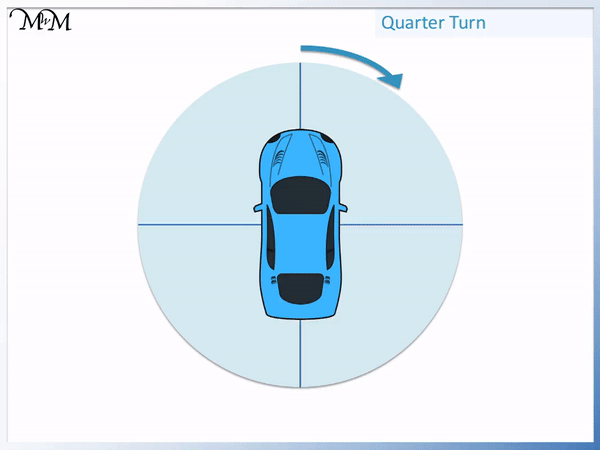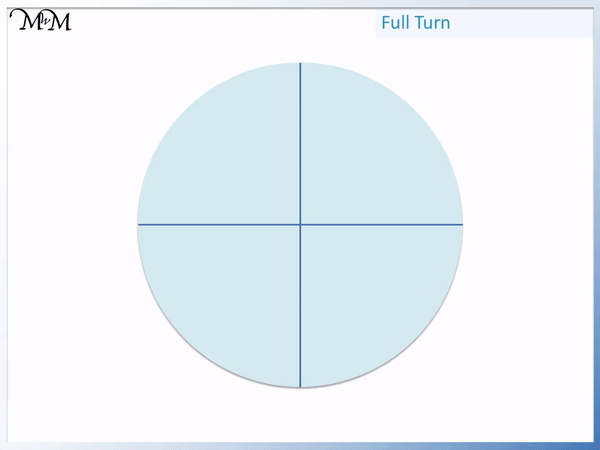# Quarter, Half and Three Quarter Turns

Quarter, Half and Three Quarter Turns• The car is resting on a circle divided into four quarters.
• The car will turn in the direction shown.
• As it reaches each new line it will have turned a quarter turn.
• As the car reaches the first line, it has turned through one of the four parts of the circle – this is a quarter turn.
• As the car reaches the second line, it has turned through two of the four parts of the circle – this is half a turn.
• After turning half a turn, the car is facing in the opposite direction to where it started.
• It then reaches the third line and has turned through three of the four parts of the circle – this is a three-quarter turn.
• The fourth line that the car reaches is back where it started – this is a full turn.

A half turn is in the opposite direction and a quarter turn is half of this.

A full turn is facing in the original direction and a three-quarter turn is half way between half a turn and a full turn.Supporting Lessons# How to Describe Position and Direction Using Quarter, Half and Three Quarter Turns

In this lesson we are introducing the concept of describing direction using turns.

There are four possible types of turn that are first taught:

1. A quarter turn
2. A half turn
3. A Three-quarter turn
4. A full turn
5. The car in the example below is resting on a circle which is divided into four equal parts.

Each of these parts are known as quarters, since they are equal in size.

We will first look at a quarter turn.In a quarter turn, one quarter of the full turn is made.

We can see that one of the four quarters of the circle has been shaded as the car rotates.

When the car is in line with the first new line that it comes to, it has made a quarter turn.

In school based questions or elsewhere, your child may not be given a circle with the lines dividing it into quarters.

When teaching quarter turns, we can draw in the quarters onto examples that do not have them to help visualise it more clearly. This can be done by drawing a simple cross.

If the object is facing upwards then a quarter turn will mean that the object is then facing left or right, depending which direction it turns.

The next turn we will look at is a half turn.

A half turn results in the object facing the opposite direction (or backwards) to the direction it was originally facing.The car was originally facing upwards and so after half a turn, it is now facing downwards.

The reason that this is half a turn is that the car has rotated through half of the circle behind it. It is halfway around the full rotation. Another of these half turns will make a whole turn or full turn.

The car has turned through two of the four quarters of the circle behind it. Two out of four is the same as one half.

The next turn we will look at is a three-quarter turn.

Each of the lines that the car gets to as it rotates is one quarter of a turn.

We can see it sweeping out one quarter of the circle as it gets to each new grid line.In a three-quarter turn, we turn through three of the four quarters.

The car rotates to be in line with the third line it comes to.

When teaching a three-quarter turn, we can count how many quarters have been shaded and show that it is equal to three. We can also show that this is opposite a one quarter turn.

The car is facing in the opposite direction to when it rotated a one quarter turn.

The car is one quarter turn away from being back to where it started.

The final turn is a full turn or whole turn.

In a full turn, the object rotates completely and is facing in the same direction to where it started.The car has turned through all four quarters.

It has turned fully.

A full turn results in no overall change to the direction of the object compared to the beginning.Now try our lesson on Naming 2D Shapes Using Sides and Corners where we learn how to find the names of 2D shapes.